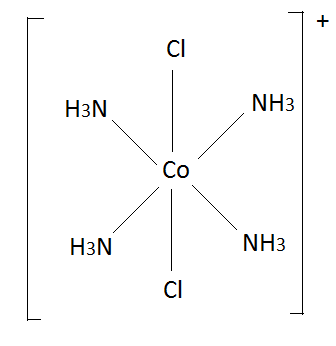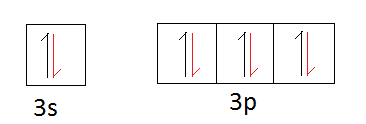# Difference Between Oxidation Number and Valency

## Main Difference – Oxidation Number vs Valency

Oxidation number and valency are related to the valence electrons of an atom. Valence electrons are the electrons that occupy the outermost shells or orbitals of an atom. As these electrons are weakly attracted to the nucleus, they can easily be lost or shared with other atoms. This loss, gain or sharing of electrons cause a particular atom to have an oxidation number and valency. The main difference between oxidation number and valency is that oxidation number is the charge of the central atom of a coordination compound if all bonds around that atom were ionic bonds whereas valency is the maximum number of electrons that an atom can lose, gain or share in order to become stable.

### Key Areas Covered

1. What is Oxidation Number
– Definition, Calculation, Representation, Examples
2. What is Valency
– Definition, Calculation, Representation, Examples
3. What is the Difference Between Oxidation Number and Valency
– Comparison of Key Differences

Key Terms: Aufbau Principle, Coordination Compound, Ionic Bonds, Octet Rule, Oxidation Number, Valence Electrons, Valency## What is Oxidation Number

Oxidation number is the charge of the central atom of a coordination compound if all the bonds around that atom were ionic bonds. Coordination complexes are almost always composed of transition metal atoms in the center of the complex. This metal atom is surrounded by chemical groups which are called ligands. These ligands have lone electron pairs that can be shared with metal atoms to form coordination bonds. After the formation of the coordination bond, it is similar to a covalent bond. This is because the two atoms in the coordination bonds share a pair of electrons, just like a covalent bond. However, the oxidation number of the central metal atom is calculated considering the coordination bonds as ionic bonds.

In order to form coordination bonds, the metal atom should have empty orbitals. Most of the transition metals are composed of empty d orbitals. Therefore, they can act as the central metal atom of coordination complexes. The oxidation number of the central atom is represented by Roman numbers. The Roman number gives the charge of the central atom, and it is included in brackets. For example, if the oxidation number of a hypothetical metal atom “M” is 3, the oxidation number is given as M(III).

Let us consider an example to find the oxidation number. The structure of a coordination ion is given below.Figure 01: trans-[CoCl2(NH3)4]+

In the above coordination ion, the overall charge is +1; therefore, the sum of the charges of the ligands and the central atom should equal +1. Typically, Chlorine atoms are -1 charged and NH3 are neutral.

+1    =      (Charge of cobalt atom) + (charge of 2 Cl atoms) + (charge of 4 NH3)

+1    =      (Charge of cobalt atom) + (-1 x 2) + (0 x 4)

Therefore,

Charge of cobalt atom     =      (+1) – {(-2) + (0)}

=     (+3)

Hence the oxidation number of Cobalt   =   Co(III)

## What is Valency

Valency is the maximum number of electrons that an atom can lose, gain or share in order to become stabilized. For metals and nonmetals, the octet rule describes the most stable form of an atom. It says that, if the number of the outermost shell of an atom is completely filled with eight electrons, that configuration is stable. In other words, if the s and p sub-orbitals are completely filled having ns2np6, it is stable. Naturally, noble gas atoms have this electron configuration. Therefore, other elements need to either lose, gain or share electrons in order to obey the octet rule. The maximum number of electrons involved in this stabilization process is called the valency of that atom.

As an example, if we consider the Silicon element, the electron configuration is 1s22s22p63s23p2. The outermost shell is n=3. The number of electrons in that shell is 4. Therefore it should obtain 4 more electrons to complete the octet. Generally, Silicon can share 4 electrons with other elements to complete the octet.

The orbital diagram of Silicon,A rearrangement of the electrons occurs before the sharing.Then the sharing of electrons occurs.In the above orbital diagram, the half arrows in red color represent the electrons shared by other elements. Since the silicon atom should share 4 electrons in order to get stabilized, the valency of silicon is 4.

But for transition metal elements, the valency is often 2. This is because the electrons are filled to the orbitals according to the energy levels of those orbitals. For example, according to the Aufbau principle, the energy of 4s orbital is lower than that of 3d orbital. Then, the electrons are first filled to the 4s orbital and then to the 3d orbital. As valency is defined for the electrons in the outermost orbital, the electrons in 4s orbital is the valency of that atom. If we consider Iron (Fe), the electron configuration is [Ar]3d64s2. Therefore the valency of iron is 2 (2 electrons in 4s2). But sometimes, the valency of iron becomes 3. This is because the 3d5 electron configuration is more stable than 3d6. Thus, removing one more electron along with 4s electrons will stabilize Iron more.

## Difference Between Oxidation Number and Valency

### Definition

Oxidation Number: Oxidation number is the charge of the central atom of a coordination compound if all bonds around that atom were ionic bonds.

Valency: Valency is the maximum number of electrons that an atom can lose, gain or share in order to become stabilized.

### Application

Oxidation Number: Oxidation number is applied for coordination complexes.

Valency: Valency is used for any element.

### Calculation

Oxidation Number: Oxidation number can be calculated considering the ligands and the overall charge of the coordination complex.

Valency: Valency can be determined by obtaining the electron configuration.

### Representation

Oxidation Number: Oxidation number is given in Roman numbers that are inside brackets.

Valency: Valency is given in Hindu-Arabic numbers.

### Conclusion

Although the definition of Valency says that it is the maximum number of electrons used in bonding, transition elements can have different valencies. This is because transition metals can be stabilized by removing different numbers of electrons. Moreover, central atoms in coordination complexes can have different oxidation numbers according to the ligands that are attached to the atom.

##### References:

1.”Oxidation Numbers.” Chemed. N.p., n.d. Web. Available here. 20 July 2017.
2.Helmenstine, Anne Marie. “What Is Valence in Chemistry?” ThoughtCo. N.p., n.d. Web. Available here. 20 July 2017.